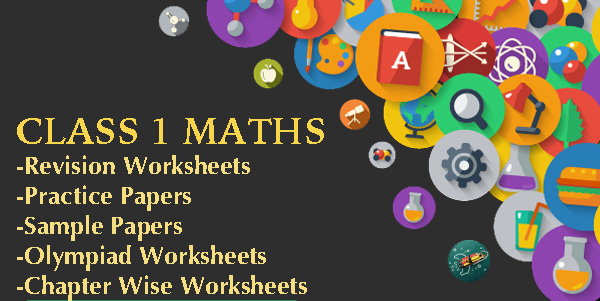CBSE Class 1 Maths Practice Worksheets and Sample PapersIn class 1, the maths curriculum is designed in an engaging and interesting way. Students are introduced to numerous important maths topics in the form of various illustrations and practical examples.CBSE Class 1 Maths Practice Worksheets and Sample Papers Play an important role in practicing and building maths fundamentals at an earlier stage. By attempting these NCERT based worksheets for Class 1 Maths is always beneficial for the students as it will boost up their confidence.These Class 1 Maths Worksheets and Papers have been uploaded by various users on ribblu.com.

Class 1 Maths Chapter wise Worksheets

```Class 1 Maths Worksheet - 3 ( Topic - Tens and Ones )

Class 1 Maths Worksheet ( Time and Addition on Number Line )

Class 1 Maths Worksheet 2019 ( MEASUREMENT )

Class 1 Maths Worksheet 2019 ( Data Handling )

Class 1 Maths Worksheet 2019 ( Money )

CLASS 1 Maths Worksheet - 1 ( Ones and Tens ) 2019

Class 1 Maths Worksheet (Time) - Air Force School

Class 1 Maths Worksheet ( Numbers upto 100 ) - Air Force School

Class 1 Maths Worksheet (Shapes & Patterns) - Air Force School```

Class 1 Maths Revision Worksheets

```Class 1 Maths Revision Worksheet - 4

Class 1 Maths Revision Worksheet – 3 (2019)

Class 1 Mathematics Revision Worksheet-2 (2019)

Class 1 MATHS REVISION WORKSHEET -1 (2019)

Class 1 Maths Worksheet - Air Force School

Class 1 Maths Worksheet (2) - Ramjas Public School

Class 1 Maths Worksheet (1) - Ramjas Public School```

Class 1 Maths Practice Papers ( Cycle tests  & Sample Papers)

```Class 1 Maths Cycle Test Paper 10 - Air Force School

Class 1 Maths Cycle Test Paper 9 - Air Force School

Class 1 Maths Cycle Test Paper 8 - Air Force School

Class 1 Maths Cycle Test Paper 1 - Air Force School

Class 1 Maths Cycle Test Paper 3 - Air Force School

Class 1 Maths Cycle Test Paper 4 - Air Force School

Class 1 Maths Cycle Test Paper 5 - Air Force School

Class 1 Maths Cycle Test Paper 6 - Air Force School

Class 1 Maths Cycle Test Paper 7 - Air Force School

CBSE Class 1 Maths Sample Paper 2 ( Annual exam ) - Prince school

Class 1 Maths Sample Paper 2 ( Half Yearly ) - Prince School```

`Class 1 International Mathematics Olympiad worksheet 1 - DPS`